# Data Science Interview Questions Data Science

Prepare better with the best interview questions and answers, and walk away with top interview tips. These interview questions and answers will boost your core interview skills and help you perform better. Be smarter with every interview.

• 4.5 Rating
• 91 Question(s)
• 30 Mins of Read

## Data Science with R

view() function show the dataset in the spreadsheet format.

Use tuneRF() function to fine-tune random forest model.

arules package is used for market basket analysisa

Correlations is produced by cor() and covariance is produced by cov() function.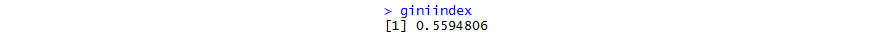Gini index is the ratio between area between the ROC curve and the diagonal line and the area of the above triangle. Gini = 2*AUC – 1. Gini above 60% is a good model.

Linear combination of independent variables explains 70% of the variance in the dependent variable. More the variance it learns, better the model is.

If mean and standard deviation is not passed to the rnorm() function, then the normal distribution has a density of mean=0 and standard deviation=1.

pnorm(q, 0,1) with mean=0 and standard deviation =1, will give you the area under a standard normal distribution curve where q is the z-score. However, the default parameter that goes into pnorm() is lower.tail=TRUE which means, pnorm(1.96, 0,1) is same as pnorm(1.96, 0,1, lower.tail=TRUE). Hence, pnorm(1.96, 0,1) gives the area under the standard normal curve to the left of 1.96. With lower.tail=FALSE, i.e. pnorm(1.96, 0,1, lower.tail=FALSE) will give you the area under the standard normal curve to the right of 1.96.

The statement is correct.

The form of the glm() function is glm(formula, family=familytype(link=linkfunction), data=). Here, familytype should be a string value “binomial” to indicate that the dependent variable is a binomial variable.

install.packages(“ggplot2”) should install the ggplot2 package. However, if you are using linux system and do not have access to the root access, this command won’t work. In order to install packages in linux system without root access, you need to designate a directory where the downloaded packages to be stored. After creating the directory, to install the package, you can use a command, for example, install.packages(“ggplot2”, lib=”/data/Rpackages/”)

t-value is calculated by dividing the Estimate of the respective independent variables by their standard errors. The standard error is an estimate of the standard deviation of the coefficient, the amount it varies across cases. It can be thought of as a measure of the precision with which the regression coefficient is measured.

Standard Error is given by

The beta coefficient and the estimate are same.A residual is a difference between true value and the predicted value. Here the max error is 9.114 indicates that the model under-predicted expenses by \$9.114 for at least one observation. On the other hand, 50% of the errors fall within 1st quartile and 3rd quartile so the majority of the predictions were between \$1.440 over the true value and \$1.473 under the true value.

Both Shapiro Wilk & Anderson Darling test can be used to test if a data is normally distributed. However, Shapiro Wilk works for a dataset where the number of observations is up to 5000. For datasets with more than 5000 observations, you may use Anderson Darling test. Breusch Pagan test is used to test heteroscedasticity.

Correlation Matrix can be generated using the cor() function in R, by passing the dataset as a parameter to the cor(). Example: cor(dataset name). However, all variables in the dataset need to be numeric/integer else the cor() will throw an error. A high correlation is indicated by numbers close to -1 or +1.

VIF is used to detect multicollinearity. The square root of the variance inflation factor indicates how much larger the standard error is, compared with what it would be if that variable was uncorrelated with the other predictor variables in the model. If the variance inflation factor of a predictor variable were 5.27 (√5.27 = 2.3), this means that the standard error for the coefficient of that predictor variable is 2.3 times as large as it would be if that predictor variable was uncorrelated with the other predictor variables.Shapiro Wilk test is used to check the normal distribution of dependent variable.

HO: Population is Normally Distributed

Ha: Population is not Normally Distributed

Here, p-value is 0.01<0.05, Hence reject HO. So we can conclude that Ha is accepted, that is Data is not normally distributed.

For instance, homeless population and crime rate might be correlated, in that both tend to be high or low in the same locations. It is equally valid to say that homeless population is correlated with crime rate, or crime rate is correlated with homeless population. To say that crime causes homelessness, or homeless populations cause crime are different statements. And correlation does not imply that either is true. For instance, the underlying cause could be a 3rd variable such as drug abuse, or unemployment which is known as confounding variable.

Yes, there can be a no intercept model where slope passes through the origin. There is no R square in no-intercept model. For example, when no chemical is added, then output is zero.

Heteroscedasticity means a variance of errors of fitted values is high. This process is sometimes referred to as residual analysis. It important to check whether the model explains some pattern in the response variable y, it can result in an inefficient and unstable regression model. It can be identified through graphical or statistical method. Let’s check with car dataset,

1.  Graphical Method: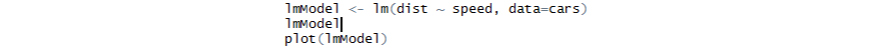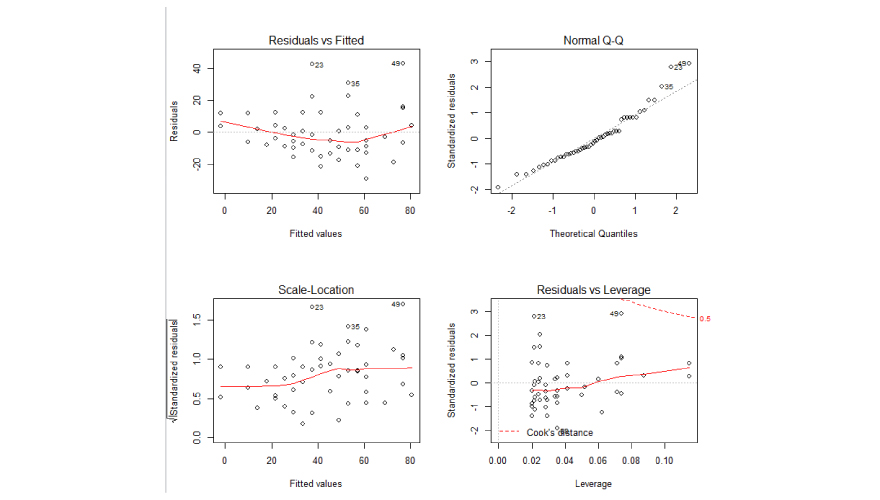In the above plot, top-left is the chart of residuals vs fitted values, while in the bottom-left one, it is standardized residuals on Y axis. If there is absolutely no heteroscedasticity, you should see a completely random, equal distribution of points throughout the range of X axis and a flat red line.

But in our case, as you can notice from the top-left plot, the red line is slightly curved and the residuals seem to increase as the fitted Y values increase. So, the inference here is, heteroscedasticity exists.

1.  Statistical Method:

The presence or absence of heteroscedasticity is identified through The Breush-Pagan test and the NCV test.HO: Data is homoscedastic

Ha: Data is heteroscedastic

Both these test have a p-value less than a significance level of 0.05, therefore we can reject the null hypothesis (H0: Homoscedasticity) that the variance of the residuals is constant and infer that heteroscedasticity is indeed present, thereby confirming our graphical inference.

Box-cox transformation is a mathematical transformation of the response variable to make it approximate to a normal distribution in order to remove heteroscedasticity.

In the example below: Initially, we apply transformation on the response variable which is shown as distBCMod and add the variable to the dataset car and new linear model lmMod_bc is generated.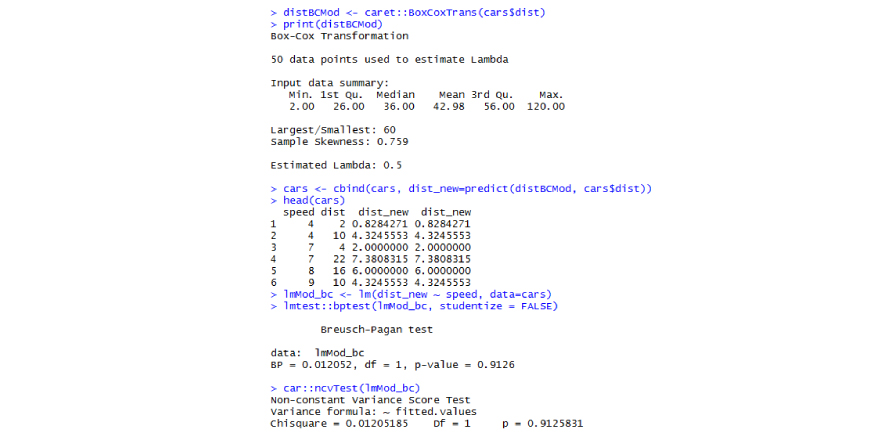After applying box-cox transformation, p-value of the Breusch-Pagan test is 0.91, hence we fail to reject the null hypothesis (that variance of residuals is constant) and therefore infer that their residuals are homoscedastic. Let’s check this graphically as well.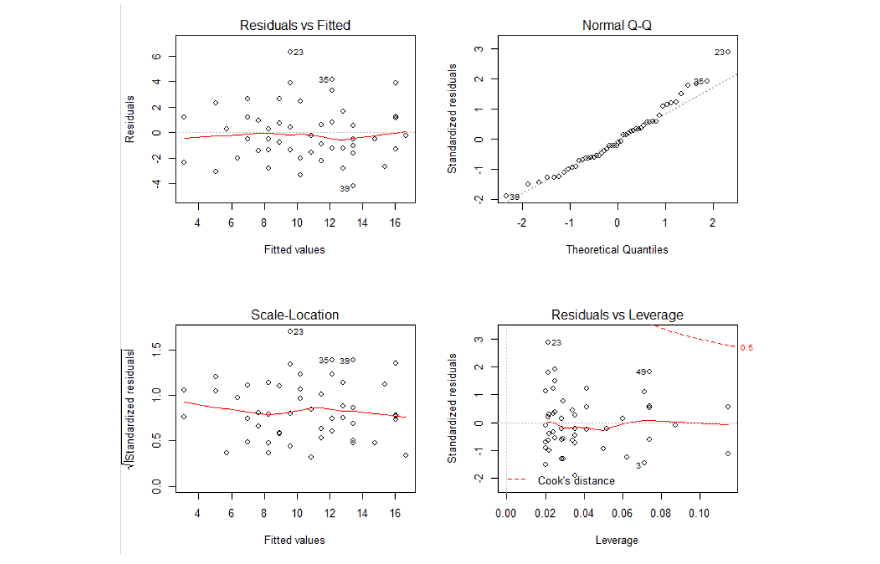We have a much flatter line and evenly distributed residuals in the top-left plot. So the problem of heteroscedasticity is solved.

Explanation: Elbow method defines clusters such that the total within-cluster sum of square (WSS) is minimized. The total WSS measures the compactness of the clustering and we want it to be as small as possible.  Steps of the algorithm are defined below,

1. Compute clustering algorithm (e.g., k-means clustering) for different values of k. For instance, by varying k from 1 to 10 clusters.
2. For each k, calculate the total within-cluster sum of square (wss).
3. Plot the curve of wss according to the number of clusters k.
4. The location of a bend (knee) in the plot is generally considered as an indicator of the appropriate number of clusters.

In R, package factoextra and Nbclust is used to perform the elbow method. For example,

Number of optimal clusters from elbow method is 4.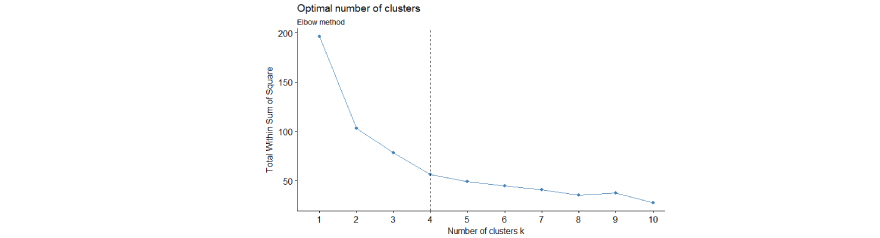Number of optimal clusters from elbow method is 4.

Standardization or Normalization is done in order to bring different scales of variables to the same scale. When the variables are in the same scale then modelling will be better. There are two methods of scaling such as Min-Max normalization and Z-score standardization. Let’s see how these methods can be applied in R. Let’s take the age and salary variables which need to be scaled.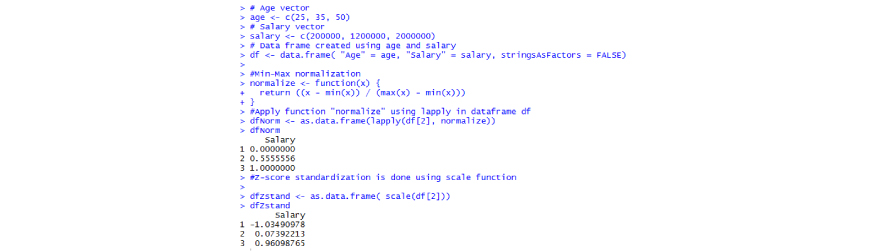geom_hline() for horizontal lines. geom_hline(yintercept, linetype, color, size) is the syntax of the function. geom_abline() for regression lines and the format is geom_abline(intercept, slope, linetype, color, size). geom_vline() for vertical lines and the format is geom_vline(xintercept, linetype, color, size).geom_segment() to add segments and the syntax is geom_segment(aes(x, y, xend, yend)).

Regression analysis requires numerical variables. So, when you wish to include a categorical variable in a regression model, you can use dummy coding in R to convert categorical into a set of separate binary variables.  In caret package, we have dummyVars function to convert categorical variables to numerical. Predict function used to add converted variables into original dataset. For example, in the energydata dataset, Orientation & glazAreaDist are categorical variables which are needed to be recoded.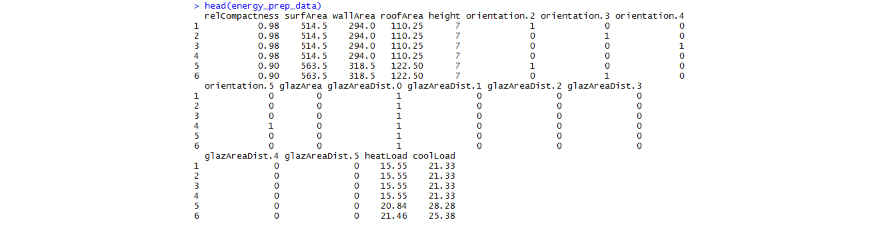Residual Deviance should be less compared to Null Deviance to show that considering independent variables actually improve the prediction of the response variable. If you have more than one similar candidate models then you should select the model that has the smallest AIC. So AIC: 696.62 are comparatively good. Fisher scoring iterations have to do with how the model was estimated. A linear model can be fit by solving closed form equations. Unfortunately, that cannot be done with logistic regression. Instead, an iterative approach (the Newton-Raphson algorithm by default) is used. The model is fit based on a guess about what the estimates might be. The algorithm then looks around to see if the fit would be improved by using different estimates instead. If so, it moves in that direction (say, using a higher value for the estimate) and then fits the model again. The algorithm stops when it doesn't perceive that moving again would yield much additional improvement. This line tells you how many iterations there were before the process stopped and output the results. Thus, the number of iterations is 5. If the number happens to be high, the model is suspect and we may not be able to make proper predictions.

It is possible for Pseudo- R2 to exceed 1 but this happens only in problematic circumstances where the residual deviance > the null deviance.  McFadden: 0.2597945, r2ML: 0.2666789(Cox and snell R squared technique), r2CU: 0.3826281(nagalkerke's R squared technique). A good model’s value lies between 0.4 and 0.6.

Receiver operating characteristic (ROCcurve shows the tradeoff between sensitivity and specificity. The closer the curve follows the left-hand border and then the top border of the ROC space, the more accurate the test. The area under the curve is a measure of text accuracy. The closer AUC for a model comes to 1, the better it is.

Correct. A scree plot displays the proportion of the total variation in a dataset that is explained by each of the components in a principal component analysis. From the scree plot, we can see that the amount of variation explained drops dramatically after the first component. To generate the scree plot, use an R function scree plot(modelname), where modelname is the name of the PCA object which can be created with princomp() function in R.

HLtest is used to measure the goodness of the fit. It measures the association between actual events and predicted probability.

HO: The model fits data well

Ha: The model does not fit data well

In HL test, null hypothesis states, the model fits data well. Model appears to fit well if we have no significant difference between the model and the observed data (i.e. the p-value > 0.05, so not rejecting the Ho). The disadvantage is, it doesn’t work well in very large or very small datasets.

A confusion matrix, also known as an error matrix, is a specific table layout that allows visualization of the performance of the model. Number 1 represents the true value and 0 represents the false value. True Positive represents 176 values, True Negative represents 46 values, False Positive represents 23 values and False Negative represents 55 values. Always False negative should be less as it creates much impact on the model.

Data(package = .packages(all.available = TRUE)) is the syntax used to list all the dataset.CRAN package repository in R has more than 6000 packages, so a data scientist needs to follow a well-defined process and criteria to select the right one for a specific task. When looking for a package in the CRAN repository a data scientist should list out all the requirements and issues so that an ideal R package can address all those needs and issues.

MaxLik is the package used for Maximum likelihood estimation. ML proceeds by creating a likelihood function L, a function of the data (y) and parameters (p).

In this case, the likelihood function is, .

To get the log-likelihood function takes logs on both sides,

l(y,p) = log(L(y,p))

= n log(p) + (T - n) log(1 - p)

When applying on R, Log-likelihood is obtained as -3.81 for the sample (0, 1, 0, 0, 1, 0) and the coding is shown below.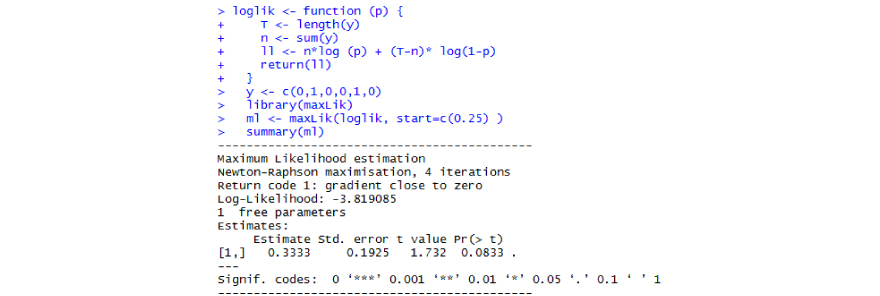t.test() is the function is used to check where the mean of the two groups are equal to each or not.

Concordance/c-statistic is a measure of the quality of fit for a binary outcome in a logistic regression model. It is a proportion of pairs in which the predicted event probability is higher for the actual event than non-event. Any value greater than 0.7 percent or 70 percent is considered a good model to use for practical purposes.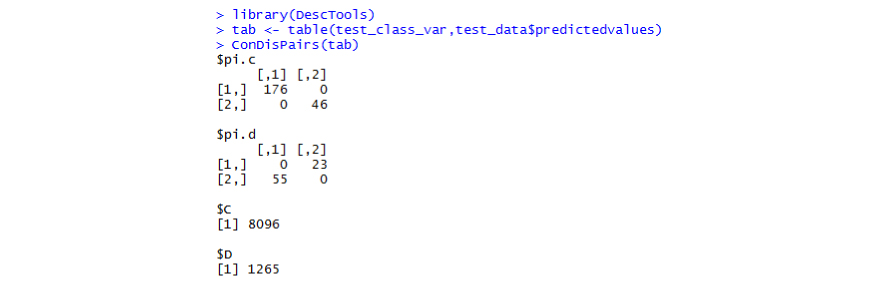rpart is based on Gini Index which measures impurity in node whereas ctree() function from "party" package uses a significance test procedure in order to select variables.

The C5.0() function makes it easy to add boost to your C5.0 decision tree. We need to add an additional "trials" parameter indicating the number of separate decision trees to use in the boosted team. The trials parameter sets an upper limit - the algorithm will stop adding trees if it recognizes that additional trails do not seem to be improving the accuracykmeans() is the function used to train the data. We know that there are 3 species involved, we ask the algorithm to group the data into 3 clusters, and since the starting assignments are random, we specify nstart = 20. This means that R will try 20 different random starting assignments and then select the one with the lowest within cluster variation.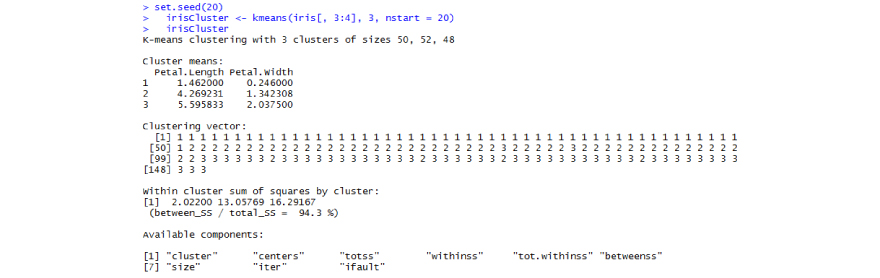The eigenvalue-one criterion, also referred to as the Kaiser criterion, is one of the methods for establishing how many components to retain in a principal components analysis. An eigenvalue less than one indicates that the component explains less variance that a variable would and hence shouldn't be retained. You can easily extract and visualize the results of PCA using R functions provided in the factoextra R package.

These functions include:

• get_eigenvalue(object): Extract the eigenvalues/variances of principal components
• fviz_eig(object): Visualize the eigenvalues
• get_pca_ind(object), get_pca_var(object): Extract the results for individuals and variables, respectively.
• fviz_pca_ind(object), fviz_pca_var(object): Visualize the results individuals and variables, respectively.
• fviz_pca_biplot(object): Make a biplot of individuals and variables.

Similarly, we can use FactoMineR package to select eigen values. The example has been shown below,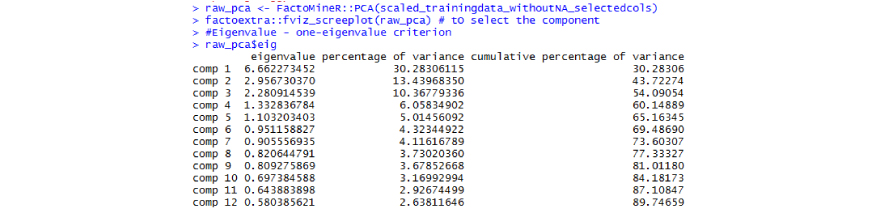The aim of principal component analysis is to explain the variance while factor analysis explains the covariance between the variables. Both Principal Components Analysis (PCA) and Factor Analysis are dimension reduction techniques. The principal Component analysis makes the components that are completely orthogonal to each other whereas Factor analysis does not require such the factors to be orthogonal i.e. the correlation between these factors is non-zero. Here is the graphic explanation, Note that the assumption is that the rectangular box contains the total variance of a model. The columns in the first figure are the features (variables) in the model. In the second figure, each colored section is a Principal Component.The factanal( ) function produces maximum likelihood factor analysis. The rotation= options include "varimax", "promax", and "none". Add the option scores="regression" or "Bartlett" to produce factor scores. Use the covmat= option to enter a correlation or covariance matrix directly.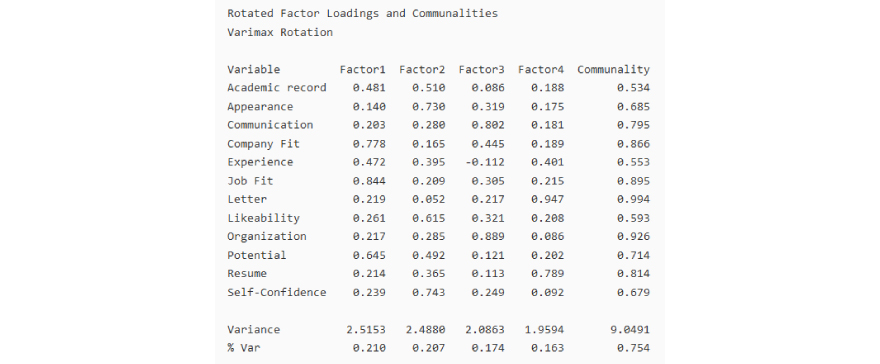In these results, a varimax rotation was performed on the data. Using the rotated factor loadings, you can interpret the factors as follows:

• Company Fit (0.778), Job Fit (0.844), and Potential (0.645) have large positive loadings on factor 1, so this factor describes employee fit and potential for growth in the company.
• Appearance (0.730), Likeability (0.615), and Self-confidence (0.743) have large positive loadings on factor 2, so this factor describes personal qualities.
• Communication (0.802) and Organization (0.889) have large positive loadings on factor 3, so this factor describes work skills.
• Letter (0.947) and Resume (0.789) have large positive loadings on factor 4, so this factor describes writing skills.

Together, all four factors explain 0.754 or 75.4% of the variation in the data.

An ANOVA(Analysis of Variance) test is a way to find out if the survey or experiment results are significant. In other words, they help you to figure out if you need to reject the null hypothesis or accept the alternate hypothesis. Basically, you’re testing groups to see if there’s a difference between them. Examples of when you might want to test different groups: Students from different colleges take the same exam. You want to see if one college outperforms the other.

aov() is the function used to find the significant difference the group. summary.aov() used to summarize the analysis of variance model. aov(formula, data = NULL, projections = FALSE, qr = TRUE,contrasts = NULL, ...) is the syntax of the model.

HO: The differences between some of the means are statistically significant

Ha: The differences between the means are not statistically significant

The p-value is lower than the usual threshold of 0.05. You are confident to say there is a statistical difference between the groups, indicated by the "*".

apriori() is the function used to perform market basket analysis.

apriori(data, parameter = NULL, appearance = NULL, control = NULL)

where parameter: The default behaviour is to mine rules with minimum support of 0.1, minimum confidence of 0.8, maximum of 10 items (maxlen), and a maximal time for subset checking of 5 seconds (maxtime). Appearance:  Appearance can be restricted. By default all items can appear unrestricted.control: Controls the algorithmic performance of the mining algorithm (item sorting, report progress (verbose), etc.)

You can do that with another library called “arulesViz” and Plot() function.

Using FSelector package, information gain can be computed. information.gain(formula, data, unit) is the syntax where the formula is a symbolic description of a model, data is the data to process and unit is the unit for computing entropy (passed to entropy). Default is "log".

stripplot() is used to understand the distribution of sepal length across all the species of flowers.

par(mfcol=c(4,3)) will ensure that the plots enter the plotting window column wise.

## Data Science with Python

When R square is 1, it means there is no error in the regression. When R square is 0, it means average line is better than the predicted line. Yes, R square can be negative when the model contains variables that do not help to predict the response variable y.

The data we receive might have missing information in specific fields. For example, the salary of a particular employee in a dataset may be missing. In that case if we perform any analysis, the result will be skewed. So it is important to have a strategy to deal with missing values.

To avoid incorrect results from any analysis, it is important to determine missing data in the dataset.  isnull() method to detect the missing values. The output shows True when the value is missing.or .isnull().sum() gives you the total number of missing values. By adding an index into the dataset, you obtain only  the entries that are missing. The example shows the following output:

0 False
1 False
2 False
3  True ( value is missing)
4 False
5 False
6  True (value is missing)

There are certain methods in dealing with missing values such as fillna(), which fills in the missing entries, When using fillna(), you must provide a value to use for the missing data.  and dropna(), drops the missing entries, Imputer (a transformer algorithm used to complete missing values). An example is shown below:

```from sklearn.preprocessing import Imputer
missing_value_df = pd.DataFrame(np.append(np.random.uniform(high=10,low=1,size=5),[np.nan,np.nan,3.2208561]),columns=['values'])```
`missing_value_df`

Values

0  4.871859

1  4.315954

2  9.013113

3  7.849918

4  4.870335

5  Nan

6  Nan

7  3.220856

`imputer = Imputer(missing_values='NaN', strategy='mean', axis=0)`
`pd.DataFrame(imputer.fit_transform(missing_value_df),columns=['values'])`

Values

0  4.871859

1  4.315954

2  9.013113

3  7.849918

4  4.870335

5  5.690339

6  5.690339

7  3.220856

One hot encoding is a process by which categorical features are converted as binary vectors. One hot encoding converts categorical feature with m possible values into m binary features.

`arr = pd.Series(['a','b','a','a','c','b'])`
`pd.get_dummies(arr)`

a b c

0  1 0 0

1  0 1 0

2  1 0 0

3  1 0 0

4  0 0 1

5  0 1 0

Hierarchical clustering involves creating clusters that have a predetermined ordering from top to bottom. There are two types of hierarchical clustering, Divisive and Agglomerative.Divisive method

In divisive or top-down clustering method we assign all of the observations to a single cluster and then partition the cluster to two least similar clusters. Finally, we proceed recursively on each cluster until there is one cluster for each observation.

Agglomerative method

In agglomerative or bottom-up clustering method we assign each observation to its own cluster. Then, compute the similarity (e.g., distance) between each of the clusters and join the two most similar clusters. The related algorithm is shown below.

Interpretation of dendrogram :-

You can find labels on the x-axis. If you don't specify anything else, they are the indices of your samples in X. You find the distances on the y-axis. (of the 'ward' which is the default one).

Summarizing dendrogram :-

• Horizontal lines are cluster merges
• Vertical lines tell you which clusters/labels were part of merge forming that new cluster
• Heights of the horizontal lines tell you about the distance that needs to be "bridged" to form the new cluster
```from matplotlib import pyplot as plt
from scipy.cluster.hierarchy import dendrogram, linkage
import numpy as np
%matplotlib inline
np.set_printoptions(precision=5, suppress=True)  # suppress scientific float notation
#Generating Sample Data
# The only thing you need to make sure is that you convert your data into a matrix X with n samples and m features, so that X.shape == (n, m).
# generate two clusters: a with 100 points, b with 50:
np.random.seed(4711)  # for repeatability of this tutorial
a = np.random.multivariate_normal([10, 0], [[3, 1], [1, 4]], size=[100,])
b = np.random.multivariate_normal([0, 20], [[3, 1], [1, 4]], size=[50,])
X = np.concatenate((a, b),)
print(X.shape)  # 150 samples with 2 dimensions

# generate the linkage matrix
Z = linkage(X, 'ward')
# calculate full dendrogram
plt.figure(figsize=(25, 10))
plt.title('Hierarchical Clustering Dendrogram')
plt.xlabel('sample index')
plt.ylabel('distance')
dendrogram(
Z,
leaf_rotation=90.,  # rotates the x axis labels
leaf_font_size=8.,  # font size for the x axis labels
)
plt.show()```

(150,2)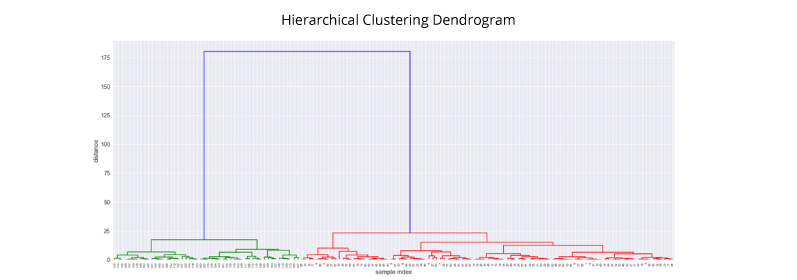You can use lambda function and specify axis = 0 lambda operator or lambda function is used for creating small, one-time and anonymous function objects in Python. lambda operator can have any number of arguments, but it can have only one expression. It cannot contain any statement and it returns a function object which can be assigned to any variable.

```from sklearn.datasets import load_iris
import pandas as pd
df = pd.DataFrame(data.data, columns=data.feature_names)
# Checking the missing value count in each column:
df.apply(lambda x: sum(x.isnull()),axis=0)
# Here we see that there are no missing values in any column of the dataset.```

sepal length (cm)    0
sepal width (cm)     0
petal length (cm)    0
petal width (cm)     0
dtype: int64

You can fill missing values with mean using the following: Let's say column 'sepal length' had missing values which we want to replace with the mean of that column df['sepal length'].fillna(df['sepal length'].mean(), inplace=True)

Option C is correct.
In Bagging, each individual trees are independent of each other because they consider different subset of features and samples.

Option B is correct.

In boosting tree, individual weak learners are not independent of each other because each tree correct the results of previous tree. Bagging and boosting both can be considered as improving the base learners results.

Sensitivity:Specificity:```# Confusion matrix creation
%matplotlib inline
import seaborn as sns
import pandas as pd
import matplotlib.pyplot as plt
from sklearn.metrics import confusion_matrix
y_actu = pd.Series([0, 1, 0, 0, 0, 1, 1, 0, 1, 0, 1, 0], name='Actual')
y_pred = pd.Series([0, 1, 1, 0, 0, 1, 1, 0, 0, 0, 1, 1], name='Predicted')
cm = confusion_matrix(y_actu,y_pred)
cm
df_cm = pd.DataFrame(cm)
df_cm.index = ['0','1']
df_cm.columns = ['0','1']
names = ['0','1']
print('confusion matrix:')
print(df_cm)```

Confusion matrix:
0  1
0  5  2
1  1  4

```cm
sensitivity = cm/(cm + cm)
sensitivity
specificity = cm/(cm + cm)
specificity
print("Sensitivity =",sensitivity,",","Specificity =",specificity)```

Sensitivity = 0.714285714286 , Specificity = 0.8

```df = pd.DataFrame([(1, 2), (0, 3), (2, 0), (1, 1)],columns=['dogs', 'cats'])
df```

Dogs     cats

0           1           2

1           0           3

2           2           0

3           1           1

`df.cov()`

Dogs              cats

Dogs           0.666667   -1.0000000

cats          -1.000000      1.6666667

Stratified sampling splits data into parts which contains approximately the same percentage of samples of each target class as the complete set. It is used for splitting your data into train or test subsets and it is also used for model selection using k fold cross validation.

Example:

```import pandas as pd
import numpy as np
from sklearn.model_selection import StratifiedKFold

```
```X = np.random.uniform(high=10,low=1,size=20)
X```

array([6.63122498, 7.64011068, 2.32874312, 2.13340531, 8.49727574,
4.33596869, 4.54053788, 3.63196184, 6.77693898, 7.94006532,
1.16825748, 7.58422557, 8.34135477, 2.69662581, 5.28611659,
2.01591238, 5.5581933 , 4.64500518, 5.16578009, 8.12041739])

```y = [0, 0, 0, 0, 1, 1, 1, 1, 1, 1, 0, 0, 1, 1, 1, 0, 0, 0, 1, 1]
startified_sample_1,startified_sample_2,startified_sample_3 = StratifiedKFold(n_splits=3).split(X,y)```
`startified_sample_1`

(array([ 3,  8, 9, 10, 11, 12, 13, 14, 15, 16, 17, 18, 19]),
array([0, 1, 2, 4, 5, 6, 7]))

`startified_sample_2`

(array([ 0,  1, 2, 4, 5,  6, 7, 14, 15, 16, 17, 18, 19]),
array([ 3,  8, 9, 10, 11, 12, 13]))

`startified_sample_3`

(array([ 0, 1,  2, 3, 4, 5, 6,  7, 8, 9, 10, 11, 12, 13]),
array([14, 15, 16, 17, 18, 19]))

Silhouette value is a measure of how similar an object is to its own cluster (cohesion) compared to other clusters (separation). The silhouette measure ranges from −1 to +1, where a high value indicates good cohesive clusters.

averageSilhouetteScore=∑Ni=1(AiBi)max(Ai,Bi)NaverageSilhouetteScore=∑i=1N(Ai−Bi)max(Ai,Bi)N

• AiAi is the average distance between i th point in a cluster with all the points in the same cluster
• BiBi is the average distance between i th point in a cluster with all the points in the other clusters
• N is the number of data points
```from sklearn.datasets import make_blobs
from sklearn.cluster import KMeans
from sklearn.metrics import silhouette_samples, silhouette_score
import matplotlib.cm as cm

```
```import matplotlib.pyplot as plt
# Generating the sample data from make_blobs
# This particular setting has one distinct cluster and 3 clusters placed close
# together.
X, y = make_blobs(n_samples=500,
n_features=2,
centers=4,
cluster_std=1,
center_box=(-10.0, 10.0),
shuffle=True,
random_state=1)```
`range_n_clusters = np.arange(2,11)`
`range_n_clusters`

array([ 2,  3,  4,  5,  6,  7,  8,  9, 10])

```# num_cluster_ls = []
# silhouette_score_ls = []
# for i in range_n_clusters:
#     clusterer = KMeans(n_clusters=i, random_state=10)
#     cluster_labels = clusterer.fit_predict(X)
#     silhouette_avg = silhouette_score(X, cluster_labels)
#     num_cluster_ls.append(i)
#     silhouette_score_ls.append(silhouette_avg)
# plt.plot(silhouette_score_ls,num_cluster_ls)
# plt.show()```
```for n_clusters in range_n_clusters:
clusterer = KMeans(n_clusters=n_clusters, random_state=10)
cluster_labels = clusterer.fit_predict(X)
# The silhouette_score gives the average value for all the samples.
# This gives a perspective into the density and separation of the formed
# clusters
silhouette_avg = silhouette_score(X, cluster_labels)
print("For n_clusters =", n_clusters,
"The average silhouette_score is :", silhouette_avg)```

For n_clusters = 2 The average silhouette_score is : 0.704978749608
For n_clusters = 3 The average silhouette_score is : 0.588200401213
For n_clusters = 4 The average silhouette_score is : 0.650518663273
For n_clusters = 5 The average silhouette_score is : 0.563764690262
For n_clusters = 6 The average silhouette_score is : 0.450466629437
For n_clusters = 7 The average silhouette_score is : 0.390922110299
For n_clusters = 8 The average silhouette_score is : 0.331485389965
For n_clusters = 9 The average silhouette_score is : 0.334343241561
For n_clusters = 10 The average silhouette_score is : 0.339292096484

From above values of silhouette score we can say that the optimal number of clusters is 2.

The Kappa statistic (or value) is a metric that compares an Observed Accuracy with an Expected Accuracy (random chance). The kappa statistic is used not only to evaluate a single classifier, but also to evaluate classifiers amongst themselves. In addition, it takes into account random chance (agreement with a random classifier), which generally means it is less misleading than simply using accuracy as a metric (an Observed Accuracy of 80% is a lot less impressive with an Expected Accuracy of 75% versus an Expected Accuracy of 50%). Computation of Observed Accuracy and Expected Accuracy is integral to comprehension of the kappa statistic, and is most easily illustrated through use of a confusion matrix. Let’s begin with a simple confusion matrix from a simple binary classification of Cats and Dogs

`pd.DataFrame({'Cats':[10,5],'Dogs':[7,8]},index=['Cats','Dogs'])`

Cats    dogs

Cats      10       7

Dogs       5       8

From the confusion matrix we can see there are 30 instances total(10 + 7 + 5 + 8 = 30). According to the first column 15 were labeled as Cats (10 + 5 = 15), and according to the second column 15 were labeled as Dogs (7 + 8 = 15). We can also see that the model classified 17 instances as Cats (10 + 7 = 17) and 13 instances as Dogs (5 + 8 = 13).

Observed Accuracy is simply the number of instances that were classified correctly throughout the entire confusion matrix, i.e. the number of instances that were labeled as Cats via ground truth and then classified as Cats by the machine learning classifier, or labeled as Dogs via ground truth and then classified as Dogs by the machine learning classifier. To calculate Observed Accuracy, we simply add the number of instances that the machine learning classifier agreed with the ground truth label, and divide by the total number of instances. For this confusion matrix, this would be 0.6 ((10 + 8) / 30 = 0.6).

Before we get to the equation for the kappa statistic, one more value is needed: the Expected Accuracy. This value is defined as the accuracy that any random classifier would be expected to achieve based on the confusion matrix. The Expected Accuracy is directly related to the number of instances of each class (Cats and Dogs), along with the number of instances that the machine learning classifier agreed with the ground truth label. To calculate Expected Accuracy for our confusion matrix, first multiply the marginal frequency of Cats for one "rater" by the marginal frequency of Cats for the second "rater", and divide by the total number of instances. The marginal frequency for a certain class by a certain "rater" is just the sum of all instances the "rater" indicated were that class. In our case, 15 (10 + 5 = 15) instances were labeled as Cats according to ground truth, and 17 (10 + 7 = 17) instances were classified as Cats by the machine learning classifier. This results in a value of 8.5 (15 17 / 30 = 8.5). This is then done for the second class as well (and can be repeated for each additional class if there are more than 2). 15 (7 + 8 = 15) instances were labeled as Dogs according to ground truth, and 13 (8 + 5 = 13) instances were classified as Dogs by the machine learning classifier. This results in a value of 6.5 (15 13 / 30 = 6.5). The final step is to add all these values together, and finally divide again by the total number of instances, resulting in an Expected Accuracy of 0.5 ((8.5 + 6.5) / 30 = 0.5). In our example, the Expected Accuracy turned out to be 50%, as will always be the case when either "rater" classifies each class with the same frequency in a binary classification (both Cats and Dogs contained 15 instances according to ground truth labels in our confusion matrix).

The kappa statistic can then be calculated using both the Observed Accuracy (0.60) and the Expected Accuracy (0.50) and the formula:

Kappa = (observed accuracy - expected accuracy)/(1 - expected accuracy)

So, in our case, the kappa statistic equals: (0.60 - 0.50)/(1 - 0.50) = 0.20

The kappa score is a number between -1 and 1. Scores above .8 are generally considered good agreement; zero or lower means no agreement (practically random labels). In python sklearn.metrics provide a function _cohen_kappa_score()_ to calculate kappa score.

`from sklearn.metrics import cohen_kappa_score`
```y_true = [1, 0, 1, 1, 0, 1]
y_pred = [0, 0, 1, 1, 0, 1]
cohen_kappa_score(y_true, y_pred)```

0.66666666666666674

```from sklearn.metrics import confusion_matrix
from sklearn.metrics import accuracy_score,f1_score,cohen_kappa_score
data = pd.DataFrame({'label':[1,1,1,1,1,1,1,1,0,0,],'predicted_label':[1,1,1,1,1,1,1,1,1,1]})```
`pd.DataFrame(confusion_matrix(y_pred=data.predicted_label,y_true=data.label))`

0     1

0    0     2

1    0     8

```print('Accuracy',accuracy_score(y_true=data.label,y_pred=data.predicted_label))
print('f1_score',f1_score(y_true=data.label,y_pred=data.predicted_label))
print('cohen_kappa_score',cohen_kappa_score(data.label,data.predicted_label))```

Accuracy 0.8
f1_score 0.888888888889
cohen_kappa_score 0.0

The Matthews correlation coefficient is used in machine learning as a measure of the quality of binary (two-class) classifications. It takes into account true and false, positives and negatives and is generally regarded as a balanced measure which can be used even if the classes are of very different sizes. The MCC is in essence a correlation coefficient value between -1 and +1. A coefficient of +1 represents a perfect prediction, 0 an average random prediction, and -1 an inverse prediction. The statistic is also known as the phi coefficient. In the binary (two-class) case, tp, tn, fp and fn are respectively the number of true positives, true negatives, false positives, and false negatives, the MCC is defined as: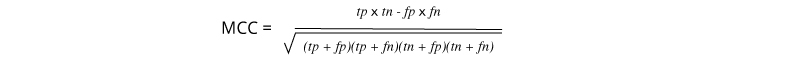```from sklearn.metrics import matthews_corrcoef
y_true = [+1, +1, +1, -1]
y_pred = [+1, -1, +1, +1]
matthews_corrcoef(y_true, y_pred)```

-0.33333333333333331

After training a scikit-learn model, it is desirable to have a way to persist the model for future use without having to retrain. We can persist a model with pickle.

```from sklearn import svm
from sklearn import datasets
clf = svm.SVC()
X, y = iris.data, iris.target
clf.fit(X, y)
import pickle
s = pickle.dumps(clf)
clf2.predict(X[0:1])```

array()

The degree of flatness or peakedness is measured by kurtosis. It tells us about the extent to which the distribution is flat or peak vis-a-vis the normal curve. The following diagram shows the shape of three different types of curves.Where,

N is total number of data points

where Xi is i-th data point

X is mean

S is standard deviation

• The distribution with kurtosis equal to 3 is known as mesokurtic. A random variable which follows normal distribution has kurtosis 3
• If the kurtosis is less than three, the distribution is called as platykurtic. Here, the distribution has shorter and thinner tails than normal distribution. Moreover, the peak is lower and also broader when compared to normal distribution.
• If the kurtosis is greater than three, the distribution is called as leptokurtic. Here, the distribution has longer and fatter tails than normal distribution. Moreover, the peak is higher and also sharper when compared to normal distribution.
```from scipy.stats import kurtosis
kurtosis([1, 2, 3, 4, 5])```

-1.3

A matrix decomposition is a way of reducing a matrix into its constituent parts. It is an approach that can simplify more complex matrix operations that can be performed on the decomposed matrix rather than on the original matrix itself.

• The LU decomposition is for square matrices and decomposes a matrix into L and U components. ie A=L.P.UA=L.P.U where P is a permutation matrix, L lower triangular with unit diagonal elements, and U upper triangular.

• The QR decomposition is for m x n matrices (not limited to square matrices) and decomposes a matrix into Q and R components A=Q.R

```from numpy import array
from scipy.linalg import lu
# define a square matrix
A = array([[1, 2, 3], [4, 5, 6], [7, 8, 9]])
print(A)```

[[1 2 3]
[4 5 6]
[7 8 9]]

```# LU decomposition
P, L, U = lu(A)```
`print(P)`

[[ 0.  1. 0.]
[ 0.  0. 1.]
[ 1.  0. 0.]]

`print(L)`

[[ 1.          0. 0.       ]
[ 0.14285714  1. 0.       ]
[ 0.57142857  0.5 1.       ]]

`print(U)`

[[  7.00000000e+00   8.00000000e+00 9.00000000e+00]
[  0.00000000e+00   8.57142857e-01 1.71428571e+00]
[  0.00000000e+00   0.00000000e+00 -1.58603289e-16]]

```## reconstructing original matrix from PLU decomposed matrix decomposed matrix
P.dot(L).dot(U)```

array([[ 1.,  2., 3.],
[ 4., 5.,  6.],
[ 7., 8.,  9.]])

```from numpy.linalg import qr
# define a 3x2 matrix
A = array([[1, 2], [3, 4], [5, 6]])
print(A)```

[[1 2]
[3 4]
[5 6]]

```# QR decomposition
Q, R = qr(A, 'complete')```
`print(Q)`

[[-0.16903085  0.89708523  0.40824829]
[-0.50709255  0.27602622 -0.81649658]
[-0.84515425 -0.34503278  0.40824829]]

`print(R)`

[[-5.91607978 -7.43735744]
[ 0.          0.82807867]
[ 0.          0.        ]]

```# reconstruct
B = Q.dot(R)
print(B)```

[[ 1.  2.]
[ 3.  4.]
[ 5.  6.]]

The cosine similarity between two vectors is a measure that calculates the cosine of the angle between them. This metric is a measurement of orientation and not magnitude.Here are two very short texts to compare

1. Julie loves me more than Linda loves me

2. Jane likes me more than Julie loves me

```# Below is term frequency matrix
X =[['me',2,2],
['Jane',0,1],
['Julie',1,1],
['Linda',1,0],
['likes',0,1],
['loves',2,1],
['more',1,1],
['than',1,1]]```
```tf = pd.DataFrame(X,columns=['Word', 'Sentence1','Sentence2'])
tf````v1,v2 = tf.iloc[:,1], tf.iloc[:,2]`
`spatial.distance.cosine(v1,v2)`

0.17841616374225089

It is a theorem that describes the result of performing the same experiment a large number of times. This theorem forms the basis of frequency-style thinking. It says that the sample mean, the sample variance, and the sample standard deviation converge to what they are trying to estimate. According to the law, the average of the results obtained from a large number of trials should be close to the expected value, and will tend to become closer as more trials are performed.

Option A is correct.

Since learning rate doesn’t affect time, so, all learning rates would take equal time.

The learning parameter only controls the magnitude of the change in the estimates.

Lower values are generally preferred as they make the model robust to the specific characteristics of tree and thus allowing it to generalize well.

The adjusted R-squared is a modified version of R-squared that has been adjusted for the number of predictors in the model.

The adjusted R-squared increases only if the new term improves the model more than would be expected by chance.It decreases when a predictor improves the model by less than expected by chance.

You use Elbow Criterion Method to determine the optimal number of clusters in kmeans.

Elbow method runs k-means clustering on a given dataset for a range of values of k (num_clusters, e.g k=1 to 5), and for each value of k, calculate sum of squared errors (SSE). The objective is to minimize SSE. The goal is to choose a small value of k that still has a low SSE, and the elbow usually represents where we start to have diminishing returns by increasing k.

The plot gives a line graph of the SSE for each value of k. If the line graph looks like an arm - a red circle in below line graph (like angle), the "elbow" on the arm is the value of optimal k (number of cluster).

```### determine k using elbow method?
from sklearn.cluster import KMeans
from sklearn import metrics
from scipy.spatial.distance import cdist
import numpy as np
import matplotlib.pyplot as plt
x1 = np.array([3, 1, 1, 2, 1, 6, 6, 6, 5, 6, 7, 8, 9, 8, 9, 9, 8])
x2 = np.array([5, 4, 5, 6, 5, 8, 6, 7, 6, 7, 1, 2, 1, 2, 3, 2, 3])
plt.plot()
plt.xlim([0, 10])
plt.ylim([0, 10])
plt.title('Dataset')
plt.scatter(x1, x2)
plt.show()
# create new plot and data
plt.plot()
X = np.array(list(zip(x1, x2))).reshape(len(x1), 2)
colors = ['b', 'g', 'r']
markers = ['o', 'v', 's']
# k means determine k
distortions = []
K = range(1,10)
for k in K:
kmeanModel = KMeans(n_clusters=k).fit(X)
kmeanModel.fit(X)
distortions.append(sum(np.min(cdist(X, kmeanModel.cluster_centers_, 'euclidean'), axis=1)) / X.shape)
# Plot the elbow
plt.plot(K, distortions, 'bx-')
plt.xlabel('k')
plt.ylabel('Distortion')
plt.title('The Elbow Method showing the optimal k')
plt.show(```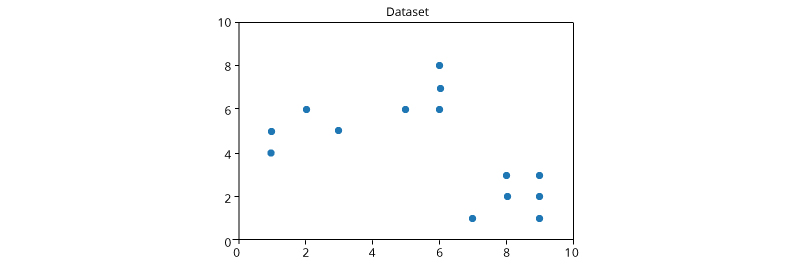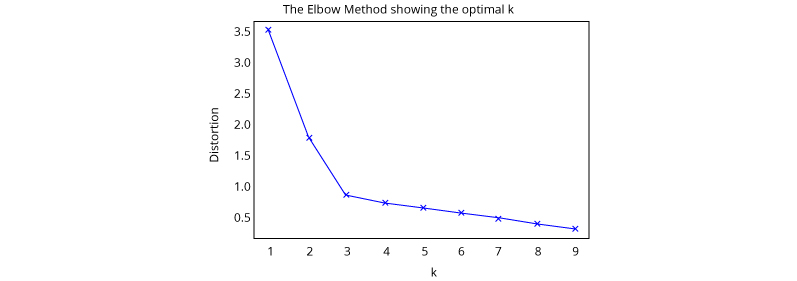Correlation is defined as covariance normalized by the product of standard deviations, so the correlation between XX and YY is defined as

Cor(X,Y)=Cov(X,Y)Var(X)Var(Y)√Cor(X,Y)=Cov(X,Y)Var(X)Var(Y)

Covariance can range between −∞−∞ and ∞∞ while correlation takes values in [−1,1][−1,1] (this is easily proved with the Cauchy-Schwarz inequality). Note that two random variables have zero correlation if and only if they have zero covariance.

A
Increasing the depth from the certain value of depth may overfit the data and for 2 depth values, validation accuracies are same; we always prefer the small depth in final model building.

A correlogram or correlation matrix allows to analyse the relationship between each pair of numerical variables of a matrix.

```# library & dataset
import seaborn as sns
import matplotlib.pyplot as plt
# Basic correlogram
sns.pairplot(df)
plt.show()
plt.close()```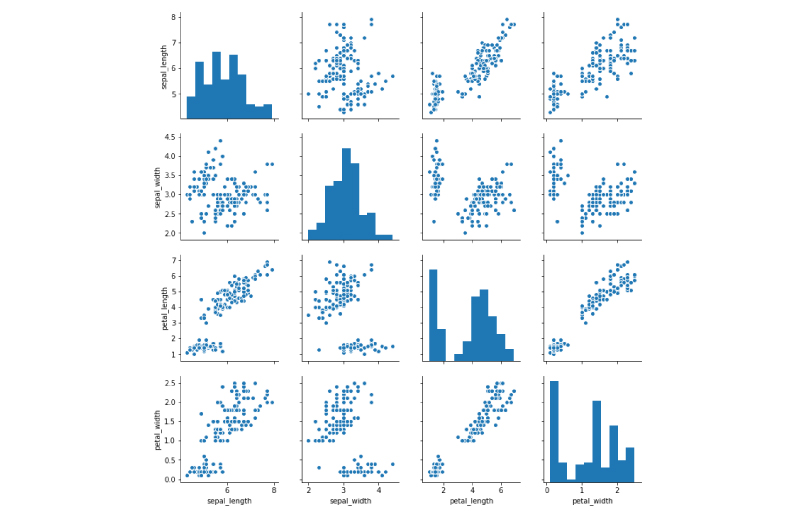Z Score method: The Z-score, or standard score, is a way of describing a data point in terms of its relationship to the mean and standard deviation of a group of points.Taking a Z-score is simply mapping the data onto a distribution whose mean is defined as 0 and whose standard deviation is defined as 1. The goal of taking Z-scores is to remove the effects of the location and scale of the data, allowing different datasets to be compared directly. The intuition behind the Z-score method of outlier detection is that, once we’ve centred and rescaled the data, anything that is too far from zero (the threshold is usually a Z-score of 3 or -3) should be considered an outlier.

Z Score method: Another robust method for labeling outliers is the IQR (interquartile range) method. A box-and-whisker plot uses quartiles (points that divide the data into four groups of equal size) to plot the shape of the data. The box represents the 1st and 3rd quartiles, which are equal to the 25th and 75th percentiles. The line inside the box represents the 2nd quartile, which is the median.

The interquartile range, which gives this method of outlier detection its name, is the range between the first and the third quartiles (the edges of the box). Tukey considered any data point that fell outside of either 1.5 times the IQR below the first – or 1.5 times the IQR above the third – quartile to be “outside” or “far out”. In a classic box-and-whisker plot, the ‘whiskers’ extend up to the last data point that is not “outside”.

```# Z Score Method
import numpy as np
def outliers_z_score(ys,threshold=1.96):
#     threshold = 3
mean_y = np.mean(ys)
stdev_y = np.std(ys)
z_scores = [(y - mean_y) / stdev_y for y in ys]
return data[np.where(np.abs(z_scores) > threshold)]
data = np.array([1, 8, 9, 10, 200])
outliers_z_score(ys=data)```

array()

```# IQR Method
def outliers_iqr(ys):
quartile_1, quartile_3 = np.percentile(ys, [25, 75])
iqr = quartile_3 - quartile_1
lower_bound = quartile_1 - (iqr * 1.5)
upper_bound = quartile_3 + (iqr * 1.5)
return data[np.where((ys > upper_bound) | (ys < lower_bound))]
data = np.array([1, 8, 9, 10, 200])
outliers_iqr(ys=data)```

array([  1, 200])

C

Bagged trees uses all the columns for only a sample of the rows. So randomization is done on the number of observations not on number of columns.

Solution is A. Conditional Probability

Only one observation is misclassified, one negative class is showing at the left side of vertical line which will be predicting as a positive class.

D

If you search any point on X1 you won’t find any point that gives 100% accuracy.

B

It is also not possible.

B

You won’t find such case because you can get minimum 1 misclassification.

A

These three examples are positioned such that removing any one of them introduces slack in the constraints.

So the decision boundary would completely change.

B

On the other hand, rest of the points in the data won’t affect the decision boundary much.

If we’re achieving 100% training accuracy very easily, we need to check to verify if we’re overfitting our data.

```mu, sigma = 0, 0.1 # mean and standard deviation
s = np.random.normal(mu, sigma, 10)

```
`s`

array([ 0.02338549, -0.02170387, -0.12129261,  0.00968304, -0.05955807,
-0.06375555,  0.06099522, -0.07360868,  0.0042497 , -0.0568088 ])

Standardization of datasets is a common requirement for many machine learning estimators, they might behave badly if the individual features do not more or less look like standard normally distributed data i.e. Gaussian with zero mean and unit variance.

StandardScaler() scales the data as per below formula: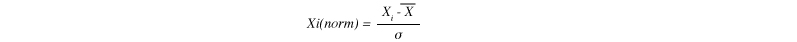MinMaxScaler() cales the data as per below formula: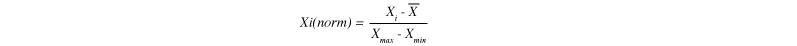```from sklearn import preprocessing
import numpy as np
X_train = np.array([[ 1., -1.,  2.],
[ 2., 0., 0.],
[ 0., 1., -1.]])

```
```stnadard_scaler = preprocessing.StandardScaler().fit_transform(X_train)

array([[ 0.        , -1.22474487, 1.33630621],
[ 1.22474487,  0. , -0.26726124],
[-1.22474487,  1.22474487, -1.06904497]])

```min_max_scaler = preprocessing.MinMaxScaler().fit_transform(X_train)
min_max_scaler```

array([[ 0.5       , 0. , 1.       ],
[ 1.     , 0.5 ,  0.33333333],
[ 0.     , 1. ,  0. ]])

The linkage criterion determines which distance to use between sets of observation. The algorithm will merge the pairs of cluster that minimize this criterion.

• Ward minimizes the sum of squared differences within all clusters.It is a variance-minimizing approach and in this sense is similar to the k-means objective function but tackled with an agglomerative hierarchical approach.
• Maximum or complete linkage minimizes the maximum distance between observations of pairs of clusters.
• Average linkage minimizes the average of the distances between all observations of pairs of clusters.

If you draw a horizontal line at 0.25 the number of intersections between x and y axis is 11 which tells you the number of clusters at that point.

3

These are the most similar points in dendrogram since they get clustered at a very small value of y axis (height).

Dendrograms work in the bottom up approach.

Bottom-up algorithms treat each document as a singleton cluster at the outset and then successively merge (or agglomerate) pairs of clusters until all clusters have been merged into a single cluster that contains all documents.

The Kruskal-Wallis H-test tests the null hypothesis that the population median of all of the groups are equal.

It is a non-parametric version of ANOVA.

The test works on 2 or more independent samples, which may have different sizes.

Note that, rejecting the null hypothesis does not indicate which of the groups differs. Post-hoc comparisons between groups are required to determine which groups are different.

```from scipy import stats
x = [1, 3, 5, 7, 9]
y = [2, 4, 6, 8, 10]
stats.kruskal(x, y)```

KruskalResult(statistic=0.27272727272727337, pvalue=0.60150813444058948)

pvalue is greater than 0.05 , hence we fail to reject the null hypothesis.

A receiver operating characteristic (ROC), or simply ROC curve, is a graphical plot which illustrates the performance of a binary classifier system as its discrimination threshold is varied. It is created by plotting the fraction of true positives out of the positives (TPR = true positive rate) vs. the fraction of false positives out of the negatives (FPR = false positive rate), at various threshold settings. TPR is also known as sensitivity, and FPR is one minus the specificity or true negative rate.

An ROC curve is a commonly used to visualize the performance of a binary classifier. It is also used to compare the performance of different models.

```import numpy as np
import matplotlib.pyplot as plt
from sklearn import svm, datasets
from sklearn.metrics import roc_curve, auc
from sklearn.model_selection import train_test_split
from sklearn.preprocessing import label_binarize
from sklearn.multiclass import OneVsRestClassifier

```
```# Import some data to play with
X = iris.data
y = iris.target
# Binarize the output
y = label_binarize(y, classes=[0, 1, 2])
n_classes = y.shape
# Add noisy features to make the problem harder
random_state = np.random.RandomState(0)
n_samples, n_features = X.shape
X = np.c_[X, random_state.randn(n_samples, 200 * n_features)]
# shuffle and split training and test sets
X_train, X_test, y_train, y_test = train_test_split(X, y, test_size=.5,
random_state=0)```
```# Learn to predict each class against the other
classifier = OneVsRestClassifier(svm.SVC(kernel='linear', probability=True,
random_state=random_state))
y_score = classifier.fit(X_train, y_train).decision_function(X_test)

```
```# Compute ROC curve and ROC area for each class
fpr = dict()
tpr = dict()
roc_auc = dict()
for i in range(n_classes):
fpr[i], tpr[i], _ = roc_curve(y_test[:, i], y_score[:, i])
roc_auc[i] = auc(fpr[i], tpr[i])```
`fpr`

{0: array([ 0.     , 0. , 0.01852,  0.01852, 0.03704, 0.03704,
0.05556, 0.05556,  0.07407, 0.07407, 0.09259,  0.09259,
0.12963, 0.12963,  0.14815, 0.14815, 0.2037 ,  0.2037 ,
0.27778, 0.27778,  1. ]),
1: array([ 0.     , 0. , 0.02222,  0.02222, 0.11111, 0.11111,
0.17778, 0.17778,  0.2 , 0.2 , 0.24444,  0.24444,
0.26667, 0.26667,  0.37778, 0.37778, 0.42222,  0.42222,
0.48889, 0.48889,  0.57778, 0.57778, 0.62222,  0.62222,
0.64444, 0.64444,  0.66667, 0.66667, 0.73333,  0.73333,
0.75556, 0.75556,  0.88889, 0.88889, 1.     ]),
2: array([ 0.     , 0. , 0.01961,  0.01961, 0.07843, 0.07843,
0.09804, 0.09804,  0.11765, 0.11765, 0.13725,  0.13725,
0.15686, 0.15686,  0.17647, 0.17647, 0.31373,  0.31373,
0.33333, 0.33333,  0.35294, 0.35294, 0.41176,  0.41176,
0.45098, 0.45098,  0.47059, 0.47059, 0.5098 ,  0.5098 ,
0.56863, 0.56863,  1. ])}

Plot of a ROC curve for a specific class

```plt.figure()
lw = 2
plt.plot(fpr, tpr, color='darkorange',
lw=lw, label='ROC curve (area = %0.2f)' % roc_auc)
plt.plot(fpr, tpr, color='red',
lw=lw, label='ROC curve (area = %0.2f)' % roc_auc)
plt.plot(fpr, tpr, color='green',
lw=lw, label='ROC curve (area = %0.2f)' % roc_auc)
plt.plot([0, 1], [0, 1], color='navy', lw=lw, linestyle='--')
plt.xlim([0.0, 1.0])
plt.ylim([0.0, 1.05])
plt.xlabel('False Positive Rate')
plt.ylabel('True Positive Rate')
plt.legend(loc="lower right")
plt.show()```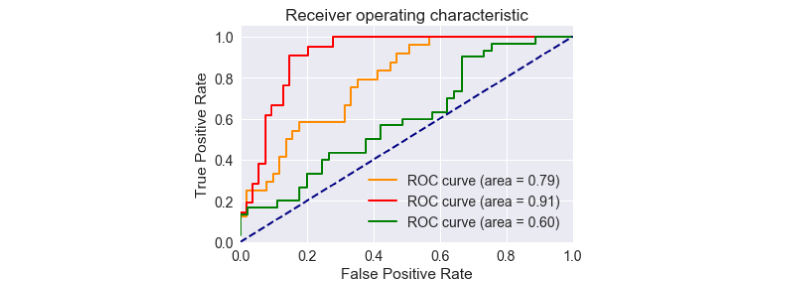The Mann-Whitney U test is a nonparametric statistical significance test for determining whether two independent samples were drawn from a population with the same distribution.

The default assumption or null hypothesis is that there is no difference between the distributions of the data samples. Rejection of this hypothesis suggests that there is likely some difference between the samples. More specifically, the test determines whether it is equally likely that any randomly selected observation from one sample will be greater or less than a sample in the other distribution. If violated, it suggests differing distributions.

• Fail to Reject H0: Sample distributions are equal
• Reject H0: Sample distributions are not equal
```from numpy.random import seed
from numpy.random import randn
from scipy.stats import mannwhitneyu
# seed the random number generator
seed(1)
# generate two independent samples
data1 = 5 * randn(100) + 50
data2 = 5 * randn(100) + 51
# compare samples
stat, p = mannwhitneyu(data1, data2)
print('Statistics=%.3f, p=%.3f' % (stat, p))
# interpret
alpha = 0.05
if p > alpha:
print('Same distribution (fail to reject H0)')
else:
print('Different distribution (reject H0)')```

Statistics=4025.000, p=0.009
Different distribution (reject H0)

The p-value strongly suggests that the sample distributions are different

Choice 4

## Description

Prepare better with the best interview questions and answers, and walk away with top interview tips. These interview questions and answers will boost your core interview skills and help you perform better. Be smarter with every interview.